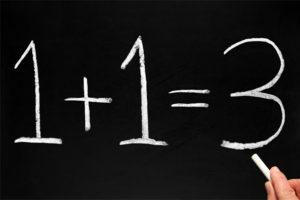Posted on

## Significant Figures and Scientific NotationFor many students, the concepts of significant figures and scientific notation are initially daunting and confusing. It is not unusual for a student to confuse the idea of rounding to a certain number of decimal places as being the same as rounding to a certain number of significant figures. Adding the concept of scientific notation into the mix can lead to the student being so uncertain as to how to write their answer for a given question.

Posted on

## Infallible Calculator?You may have seen the claim on the Internet that millions of people get the answer to the equation$6 \div 2\left(1 + 2\right)$  (or similar) wrong. You may have even read the explanations by some people about why the answer should be 9 or should be 1. Let me clear it up and explain why the answer is most definitely 1, but why the calculator (and others) give 9.

Firstly, the important thing to recognise is that this equation, like all others, is subject to the Order of Operations, which states that we must solve the contents of the brackets first. Thus our equation becomes$6 \div 2(3)$ .

Posted on

## Proof that 1 + 1 = 1. Not 2!?!Here is a quick demonstration of what can go wrong when you violate the rules of mathematics.

1. Let$a = 1$  and$b = 1$ .